# Power Pi

I was asked recently how people calculate PI. That is, what method is employed on a supercomputer to get the value of PI to a specific number of decimal places. I didn’t know off-hand, so I looked it up. Wolfram gives 32 power expansions for PI. For those of you unfamiliar with the math, a power expansion (or power series) is just another way to write out a number as the summation of individual arithmetic operations (i.e. – terms).

The simplest one to implement in VBScript is:
pi = 4 * sum [(-1)^k / (2*k + 1)] where k goes from 0 to infinity in steps of 1.

The (-1)^k part causes each iteration of the formula to oscillate between two points that gradually close in on the correct value. Then the 1/(2*k+1) is the portion of the power expansion that actually contributes to the final value. It’s easy to write, but it is slow. On my laptop, I need to run k up to 5,000,000 terms, and it takes 15 seconds to finish. Even then, the result is only accurate to 6 decimal places.

I tried to streamline the program a bit, so the part where I multiply the result by 4 isn’t until the final wscript.echo statement. Note also that there’s little point in calculating (-1)^k EVERY SINGLE TIME, so I just use sn2 to hold the current value of the sign flipping component.

Lastly,
for k=0 to 500
tmp = sn2/(2*k + 1)
next

is the same as

for k=1 to 1001 Step 2
tmp = sn2/k
next

but is maybe 25% faster to run.

res = 0
sn = -1
sn2 = 1
max = 2 * 5000000
for k = 1 to max Step 2
tmp = sn2 / k
res = res + tmp
sn2 = sn2 * sn
next

wscript.echo “res = ” & cstr(4 * res) & ” term ” & cstr(k) & ” = +/-” & cstr(2 * tmp)

The actual value of PI is: 3.14159265359
My value is 3.14159285359 At step 5,000,000
and 3.14159245359 At step 5,000,001
So, I’m at +/- 0.000000199999.

Not a really useful program, but it is fun to see the answer coming out close.

—-

There’s a more direct method with just two terms (using Excel expressions):

pi = 4 * (4 * atan(1/5) – atan(1/239))

This of course now involves finding the irrational values of two separate numbers to whatever accuracy you’re seeking for pi… Maybe not an improvement.

—-

Many of the formulas described at the Mathworld page are circular, in that to find pi, you have to use pi within the power series. Which to me seems to be kind of hard to do in software. However, I did like the version which comes from the special value of the Riemann zeta function for (pi^2)/6 = zeta(2n) for all positive integers n:

pi = sqrt(6*(1 + 1/2^2 + 1/3^2 + 1/4^2 + …))

In VBScript, this becomes:

t = 0
for n = 1 to 5000000
t = t + 1/n^2
next
wscript.echo “pi = ” & cstr((6 * t)^0.5)

pi = 3.14159246260382,

This is 29% faster than the first program above (5 seconds for 500 million terms, compared to 6.5 seconds for the first program), and they are both still accurate to only 6 places. (If both programs run for 500 million terms, the first program takes 357.6 seconds, and the second for 255.7 seconds, giving program #2 a 28.6% speed advantage, although #2 was only accurate to 7 places and #1 was already accurate to 8 places.)

After 500 million terms:
PI = 3.14159265359
#1 = 3.14159265158926
#2 = 3.14159264498239

# Back to Riemann, Part 19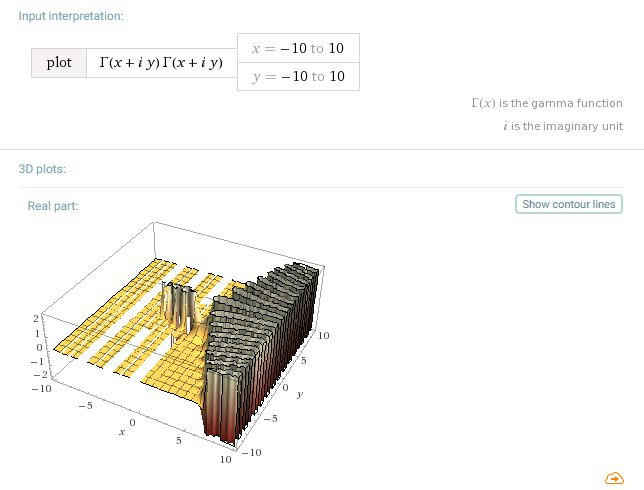(plot[gamma(x + i*y) * gamma(x + i*y) x=-10 to 10 y=-10 to 10])

Right after writing up Part 18, I returned to reading the Martin Gardner math recreations book I’d received for Christmas (it’s a big book). There were a couple articles on fractals and fractal sound, and I got to thinking that I’d like to do something similar. The key element to the Mandelbrot fractals is that they’re based on reiterations of equations on the complex plane, and that’s exactly what the gamma function does, so, who knows, maybe performing gamma on itself in some permutation might be interesting.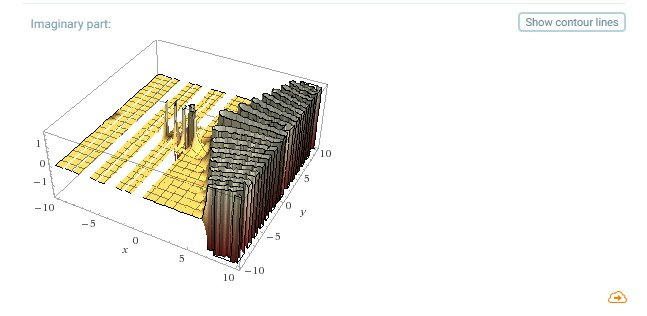After spending several days waiting for Excel to slowly grind through each of the animation approaches I decided to try, I gave up. The main problem is that the numbers start out really small and then get extremely big very fast, causing Excel to clip out most of the plots in half of the frames (even when treating the values as logs). So, from an animation viewpoint, gamma has its limitations. But, the Wolfram Alpha plots look interesting, so I’m posting them here.(Looks like a Nazca drawing.)(plot[gamma(x + i*y) * gamma(10-x + i*y)) x=-10 to 10 y=-10 to 10])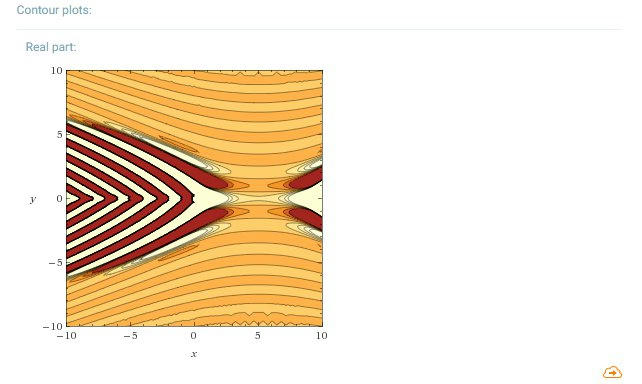(plot[gamma(x + i*y) * gamma(x + i*(10-y)) x=-10 to 10 y=-10 to 10])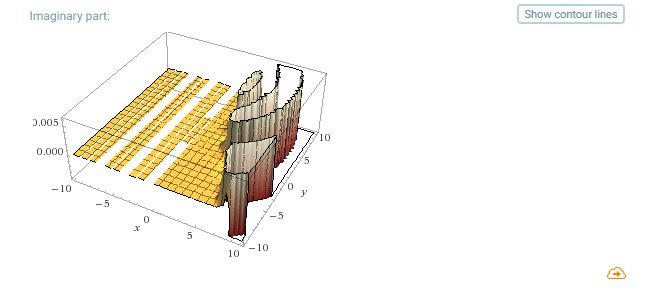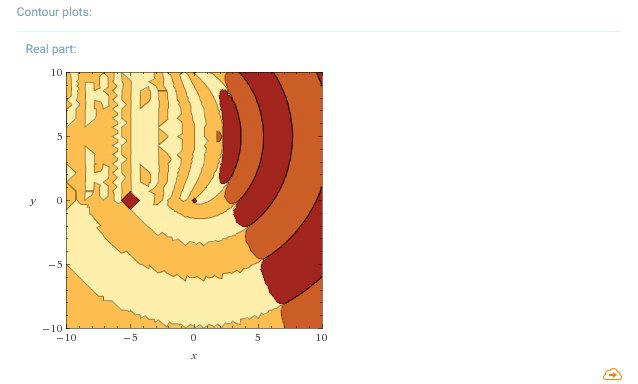(plot[gamma(x + i*y) * gamma(10-x + i*(10-y)) x=-10 to 10 y=-10 to 10])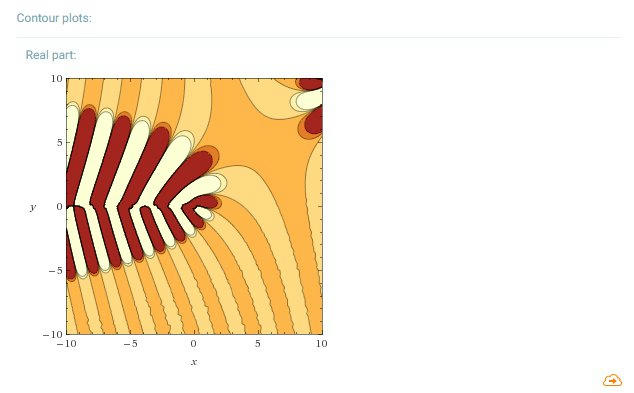(plot[gamma(gamma(x + i*y))) x=-10 to 10 y=-10 to 10])

With gamma of gamma, Wolfram actually times out. Either it draws only the real component, the real and imaginary components, or just the real contour map. The graph takes too long for it to do all four plots.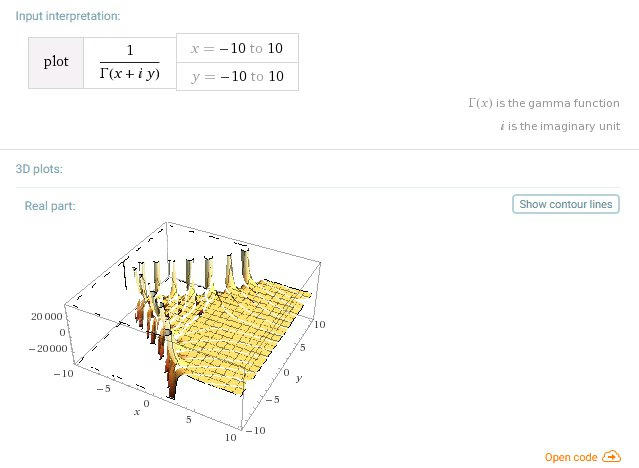(plot[gamma(1/gamma(x + i*y)) x=-10 to 10 y=-10 to 10])And with this, I’m going to call it quits for gamma for a while.

# Back to Riemann, Part 18

So, what does the gamma function look like in Wolfram Alpha?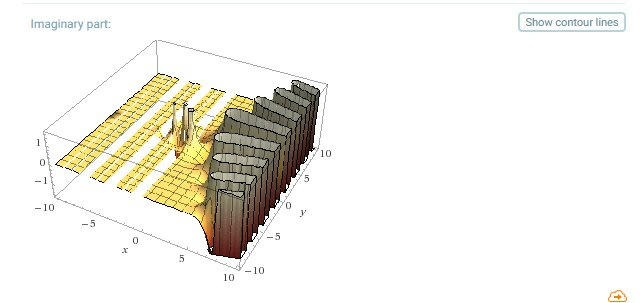From an Excel animation viewpoint, the really interesting part of the graph is when you get to about x=6. From the above plots, you can see that there are a couple small poles in the x< region, then the big one at x=0, which each provide a little activity leading up to x=6, but otherwise, the map is flat for the left hand half of the complex plane.The animation also depends on which variable you treat as the line segment. In the first pass, the segment for x=-10 to 10 was calculated for varying values of y (for y=-10 to 10). In the second pass, it was the other way around. The second animation is much more interesting. I also zoomed in on the segment for the area from -0.5 to 1. Note that for the animation where the y segment is moved around, the segment disappears due to clipping as the results from gamma() get larger. The unraveling linked spirals are kind of hypnotic, but there aren’t quite enough data points between x=6 and x=8 to generate enough frames for looping them in movie maker.

And this is pretty much where I’m going to call it quits with gamma and zeta.

A final few words on the book itself. The Riemann Hypothesis, by der Veen and de Craats is a short book, at only 144 pages, but it is highly readable, and much more approachable than any of the sources I looked at. They include a lot of exercises that can help you link the different concepts together, as well as showing how to use Wolfram Alpha for doing the computer grunt work. I don’t know why it’s suddenly so expensive on Amazon, but if you can find a copy used for \$15, it’s worth the price. Recommended if you want a decent introduction to Riemann and the zeta function. I’ve gotten about 3 month’s worth of activities from it, which isn’t bad for a little book like this.

# Back to Riemann, Part 17

Where did I leave off? Oh yeah. Despair, despair. Woe is me, I can’t figure out complex logs because the code is too complex.

Yeah, well, whatever. Turns out that if you dig enough, and use the right magic search words, you can eventually find anything on the net (maybe. I haven’t tried ALL the magic search words yet.)

What I did find pointed me back to one specific part of the wiki page on complex logs, which had this little bit of info:

log(a + bi) = ln(sqrt(a^2 + b^2)) + i*atan2(b, a)

Ok, this is good, this is good. Shame that VBScript doesn’t support atan2. atn, yes, but not atan2.

So, what is atan2 now?

Turns out that when you take the arc tangent of a complex number, say (-1 – i*1), the result is the same as for 1 + i*1. This is a problem. It’s caused by the calculation:

b/a

which wipes out the signs of the numerator and denominator if they are both negative. Plus you get a divide by zero error if a is 0.

atan2 is a function implemented in many computer languages to “correctly” calculate atan.

I found an implementation for atan2 for vbscript at www.paulsadowski.com/wsh/suntimes.htm, which in turn was lifted from a techtips page. Unfortunately, this bit of code added the result of atn() to pi, and in some cases the final answer was off by that value of pi. If you plot the results on a graph, the extra rotation gets you into the correct quadrant, but when feeding the numbers into the function for complexLog(), it screwed things up. Also, the answers weren’t corresponding to the numbers from wolfram alpha. So, I made a couple tweaks to the code to align it with wolfram.

Function atan2(ys, xs)
‘ from the Draw Arrows tip
‘ @ http://www.devx.com/upload/free/features/VBPJ/techtips/techtips11.pdf
‘ by A-Jim Deutch, Syracuse, New York
‘ I got this from http://www.paulsadowski.com/wsh/suntimes.htm – CHH

Dim theta
If xs <> 0 Then
theta = Atn(ys / xs)
If xs < 0 and ys > 0 Then
theta = theta + pi
Elseif xs < 0 and ys < 0 Then
theta = theta – pi
End If
Else
If ys < 0 Then
theta =  (-1) * pi / 2  ‘ 270
Else
theta = pi / 2          ‘ 90
End If
End If
atan2 = theta

End Function

Then, complexLog() is simply:

Function complexLog(c)
dim cplx(2)

cplx(re) = log(complexMag(c))
cplx(im) = atan2(c(im), c(re))
complexLog = cplx
End Function

From where I left off,

The real guts of the Python implementation of the Lanczos approximation is this part here:

“result = sqrt(2*pi) * t**(z+0.5) * exp(-t) * x”

I need to break this down into pieces.

From what I’ve learned about Python, exp(-t) is the same as e^(-t).
VBscript doesn’t know what “PI” and “e” are, so I have to create them as constants.
Also, since sqrt(2*pi) doesn’t change, I store this as a constant, too.

pi = 3.1415926535897932384
e  = exp(1)
sqrt2PI   = (2*pi)^0.5

And I can build up the entire product with:

t       = complexSum(z, lenAry(p) – 0.5)
minusT  = complexProd(-1, t)
t2z05   = complexPPower(t, complexSum(z, 0.5))
result1 = complexProd(t2z05, sqrt2PI)
result2 = complexProd(result1, x)
result3 = complexProd(result2, complexSPower(e, minusT))

Which leaves me with “t**(z+0.5)”, which is the Python version of “t^(z+0.5),” which I could handle as:

temp = complexSum(z, 0.5)
t2z05 = t^temp

Now, I expect that pretty much everyone has noticed that I give away part of the answer in the above snippet, where I refer to the new VBscript function complexPPower:

t2z05   = complexPPower(t, complexSum(z, 0.5))

From the mathfacility site,
f(z) = z^c = exp(c * log(z))

‘ p1^p2 = exp(p2 * log(p1))
Function complexPPower(p1, p2)
cPPT1   = complexLog(p1)
cPPT2   = complexProd(p2, cPPT1)
complexPPower = complexSPower(e, cPPT2)
end Function

And, yeah, that’s all there is to it. So, I have all this code together, and the time comes to run it. I have some syntax errors, but I fix those eventually. The code runs, and I get an answer out. I compare it to wolframalpha.com, for gamma(0.6 + i*0.4), and the numbers don’t match.

I break the code apart into individual components. I print the components out with wscript.echo. I tweak a couple lines where I made more mistakes. The numbers still don’t come out right. I put the code away for 2 weeks and play Legend of Zelda. I finish Zelda, and break the code out again. I start verifying the values generated from the for-loop on each of the values from the p[] array, and they look fine. I sum up the values from the for-loop, and they look ok. I get to the part where I apply the complex log function on “t”, and suddenly I notice something.

The code I lifted for calculating complex logs has a minus sign in front of the part that calculates the imaginary component of the result. And my values for ln(z + 0.5) have result(im) coming out negative, as they should, but the value from wolfram alpha is positive.

I change my code to remove the minus sign, and lo and behold, my results for Gamma(0.6, 0.4) are an EXACT MATCH for wolfram alpha’s!!!

I run a few more test numbers, EXACT MATCHES.

I clean up my code, add more comments, and add the code for reflection at the end when z(re) < 0.5. I run more tests with gamma(-0.413, 0.62), and the like. EXACT MATCHES.

So, yeah, I’m getting giddy now. But, why not? My code works. It may not be readable to anyone else. It may not be useful to anyone else either, including me. But, this was supposed to be a learning exercise, and I learned a lot. I can’t ask for anything more than that. And the CODE WORKS. Heh heh heh. I think I may try doing an Excel animation anyway, if I can find part of the graph that looks interesting enough.

Here’s the VBscript code, minus the comments and extra complex functions.

Dim p, epsilon, pi, e, isReflect, re, im, sqrt2PI

re        = 0
im        = 1
epsilon   = 0.0000001
pi        = 3.1415926535897932384
sqrt2PI   = (2*pi)^0.5
e         = exp(1)

p  = Array( 676.5203681218851,        -1259.1392167224028,          771.32342877765313, _
-176.61502916214059,          12.507343278686905,         -0.13857109526572012, _
9.9843695780195716e-6,      1.5056327351493116e-7)

gammaVal = gamma(complex(-0.6, 0.4))

wscript.echo “gamma (re) = ” & gammaVal(re)
wscript.echo “gamma (im) = ” & gammaVal(im)

Function gamma(zG)
isReflect = False
z         = zG

if complexReal(z) < 0.5 then
isReflect = True
z         = complexProd(-1, z)
z         = complexSum(1, z)
end if

z = complexSum(z, -1)
x = complex(0.99999999999980993, 0)

for cntr = 0 to ubound(p)
tSum     = complexSum(z, cntr + 1)
tRecip   = complexRecip(tSum)
tProduct = complexProd(p(cntr), tRecip)
x        = complexSum(x, tProduct)
next

‘  result = sqrt(2*pi) * t**(z+0.5) * exp(-t) * x
t       = complexSum(z, lenAry(p) – 0.5)
minusT  = complexProd(-1, t)
t2z05   = complexPPower(t, complexSum(z, 0.5))
result1 = complexProd(t2z05, sqrt2PI)
result2 = complexProd(result1, x)
result3 = complexProd(result2, complexSPower(e, minusT))

if( Not isReflect ) then
result  = result3
else
reflSin   = complexSin(complexProd(pi, zG))
reflect1  = complexProd(reflSin, result3)
reflRecip = complexRecip(reflect1)
result    = complexProd(pi, reflRecip)
end if

if( withinepsilon(complexImg(result)) ) then
result(im) = 0
end if

gamma = result

End Function

One last comment. The reason for storing the first value of gamma to result3, and then doing the silly bit in the if-statement to change it to result, and then later storing result to gamma is because of a limitation in VBscript. The way to return a value from a function to the caller is shown in the last line of the function.

gamma = result

This is because the name of the function is “gamma()”. Now, after storing the result to the variable gamma, any further attempts to manipulate that variable will result in syntax errors. This means that I can’t do the withinepsilon() test on the variable “gamma”. Instead, I have to do the silly bit of storing the value to “result” first if there’s no reflection, or calculate the reflection and store that to “result”, then do the withinepsilon() test of “result”. After zeroing result(im), I can finally do:

gamma = result

And not get those pesky syntax errors.

# Back to Riemann, Part 16

Back to the gamma function. There are a few more VBScript functions I have to add to my file. First, complex exponentials. From Part 13 of the blog we have:

‘ n^x = (n^a)) * (cos(b * ln(n)) – i*sin(b * ln(n))
‘ for a scalar value to a complex power
Function complexSPower(cPn, c)
dim cplx(2)                         ‘ where n = cPn is real only

cplx(re) =  cPn^(c(re)) * cos(c(im) * log(cPn))
cplx(im) =  cPn^(c(re)) * sin(c(im) * log(cPn))
complexSPower = cplx
end Function

Then, reciprocals, again from part 13, multiplying the top and bottom by (a – bi):

‘ 1/(a + bi) = (a – bi)/(a^2 – b^2)
Function complexRecip(c)
dim cplx(2)

cplx(re) =    c(re) / (c(re)^2 + c(im)^2)
cplx(im) = – (c(im) / (c(re)^2 + c(im)^2))
complexRecip = cplx
end Function

And this is where I ran into a road block with taking exponential complex numbers (i.e. – (a + bi)^(c + di)). The above code for complexSPower() takes a complex exponent of a scalar, and that’s fine. But, there’s one part of the gamma approximation that requires the power of a complex number, and I had problems finding other ways to approach this.

The issue revolves around taking the log of a complex number (ln(a + bi)). Remembering that n^x = (n^a)) * (cos(b * ln(n)) – i*sin(b * ln(n)), substituting c + di for n sticks us with (c + di)^a, and ln(c + di). Yes, I can get this far, but…

The issue is with the fact that e^pi*b*i describes a unit circle. As b increases and represents multiple integer values of pi, the circle repeats. So, the inverse function, the natural logarithm, ln(x), has an infinite number of solutions. There are several approaches to solve for ln(), but I had difficulty understanding them, or translating them into VBScript functions.

Ignoring this for the moment, let’s look at the wiki article Lanczos approximation Python code in detail (which I’m learning from scratch as I go along…)

“def gamma(z):”

This is the start of a function definition. In VBScript, this is:

Function gamma(zG)
z = zG

gamma = result
End Function

Then we have:

“epsilon = 0.0000001
def withinepsilon(x):
return abs(x) <= epsilon”

This defines a constant, called epsilon. It’s followed by inline code defining a function for testing whether the absolute value of the imaginary part of z is less than epsilon. If it is, the imaginary part will be discarded later on. In VBScript, this is:

epsilon       = 0.0000001
Function withinepsilon(we)
withinepsilon = abs(we) <= epsilon
end Function”

Then we get the import statement:

“from cmath import sin,sqrt,pi,exp”

which doesn’t have a VBScript equivalent.

And,

“p = [ 676.5203681218851,   -1259.1392167224028,  771.32342877765313,
-176.61502916214059,     12.507343278686905, -0.13857109526572012,
9.9843695780195716e-6, 1.5056327351493116e-7]
z = complex(z)”

Here, we’re creating an array of the pre-created constants for Ag(z), and a re-cast that forces the z passed to the gamma(z) function to a complex number. My version in VBScript is:

p = Array( 676.5203681218851,        -1259.1392167224028,          771.32342877765313, _
-176.61502916214059,          12.507343278686905,         -0.13857109526572012, _
0.0000099843695780195716,     0.00000015056327351493116)
z     = complex(0.6, 0.4)

Where I’m just picking 0.6 and 0.4 arbitrarily as test numbers for debugging the program.

Now, the reflection formula is tied to the fact that the Lanczos approximation only holds for z >= 0.5. If z < 0.5, which is true for the entire left side of the complex plane for z(re) < 0, then we can apply a conversion formula,

pi / (sin(pi*z) * gamma(1-z))

Python supports recursion, where you can call a function from within itself. VBScript can’t do this. Instead, I have to break the code up into two parts, and set a flag:

isReflect = False
pi        = 3.1415926535897932384

if complexReal(z) < 0.5 then
isReflect = True
z         = complexProd(z, -1)
z         = complexSum(1, z)
end if

What I’m doing is using my complex product and summation functions to calculate z = 1 – z.
(I could also do z = complexSum(1, complexProd(-1, z)).)
I then apply the following gamma code to the new value. Afterward, I use something like:

if( Not isReflect ) then
gamma     = result3
else
reflSin   = complexSin(complexProd(pi, zG))
reflect1  = complexProd(reflSin, result3)
reflRecip = complexRecip(reflect1)
reflect2  = complexProd(pi, reflRecip)
gamma     = reflect2
end if

Or,

gamma = complexProd(pi, complexRecip(complexProd(complexSin(complexProd(pi, zG)), result3)))

Where result3 is the value calculated for gamma(1 – z).
I’ll discuss this in the next blog entry.

The rest of the Python code continues in a fairly straightforward manner:

“z -= 1
x = 0.99999999999980993”

Ok, fine. In VBScript,

z = complexSum(z, -1)
x = complex(0.99999999999980993, 0)

Then comes a kind of tricky part:

“for (i, pval) in enumerate(p):
x += pval/(z+i+1)”

Python is stepping through the p list one element at a time to build up the value for Ag(z), adding each new result to x. I don’t really like the Python use of “i” as a variable in the for-loop because I keep confusing it with i as sqrt(-1). I changed it to cntr. I can do this as:

for cntr = 0 to ubound(p)
tSum     = complexSum(z, cntr + 1)
tRecip   = complexRecip(tSum)
tProduct = complexProd(p(cntr), tRecip)
x        = complexSum(x, tProduct)
next

The reason for breaking each part of the equation out and giving them unique names is that it makes things easier for debugging, and printing out the values of each step if needed.
I.e. – (within the for-loop)

if(1 = 0) then
wscript.echo “cntr                  = ” & cntr
wscript.echo “(z + cntr + 1) (re)   = ” & tSum(re)
wscript.echo “(z + cntr + 1) (im)   = ” & tSum(im)
wscript.echo “1/tSum (re)           = ” & tRecip(re)
wscript.echo “1/tSum (im)           = ” & tRecip(im)
wscript.echo “p(cntr)               = ” & p(cntr)
wscript.echo “tRecip * p(cntr) (re) = ” & tProduct(re)
wscript.echo “tRecip * p(cntr) (im) = ” & tProduct(im)
wscript.echo “x += tTemp (re)       = ” & x(re)
wscript.echo “x += tTemp (im)       = ” & x(im)
end if

Or, in one line, I’d use:

for cntr = 0 to ubound(p)
x      = complexSum(x, complexProd(p(cntr), complexRecip(complexSum(z, cntr + 1))))
next

Now, another part of the Python code that I’m not happy with is the use of len(p) on the list to get the total number of elements inside it.

“t = z + len(p) – 0.5”

This introduces a new glitch, because the VBscript equivalent, ubound(), returns the highest subscript of the array, not the total number of elements in it. ubound(p) = 7, and the Python len(p) = 8. I created a new function to address this.

Function lenAry(lA)
lenAry = ubound(lA) + 1
End Function

Which gives me:
t = complexSum(z, lenAry(p) – 0.5)

Eventually, I’m going to need -t, so I do it this way:

minusT  = complexProd(-1, t)

The real guts of the Lanczos approximation is this part here:

“result = sqrt(2*pi) * t**(z+0.5) * exp(-t) * x”

Let me skip over that for a second and look at the last part of the Python code:

“if withinepsilon(result.imag):
return result.real
return result”

All this says is that if the imaginary part of the calculation results is really small, punt and say that gamma is just comprised of the real part. Otherwise, return the calculation result. My version is:

if( withinepsilon(complexImg(result)) ) then
result(im) = 0
end if

gamma = result
End Function

Ok, the gamma guts.

“result = sqrt(2*pi) * t**(z+0.5) * exp(-t) * x”

I need to break this down into pieces.

From what I’ve learned about Python, exp(-t) is the same as e^(-t).
VBscript doesn’t know what “PI” and “e” are, so I have to create them as constants.
Also, since sqrt(2*pi) doesn’t change, I store this as a constant, too.

pi = 3.1415926535897932384
e  = exp(1)
sqrt2PI   = (2*pi)^0.5

And I can build up the entire product with:

t       = complexSum(z, lenAry(p) – 0.5)
t2z05   = complexPPower(t, complexSum(z, 0.5))

minusT  = complexProd(-1, t)
e2nT   = complexSPower(e, minusT)

result1 = complexProd(t2z05, sqrt2PI)
result2 = complexProd(result1, x)
result3 = complexProd(result2, complexSPower(e, minusT))

Which leaves me with “t**(z+0.5)”, which is “t^(z+0.5)”, and would be the same as:

temp = complexSum(z, 0.5)
t2z05 = t^temp

And this is where I bog down because t is a complex number, and I don’t know how to take ln(t).

As I said, the code is getting clunky already, and adding a function for taking the powers of a complex number is only going to make it worse.

The other thing is that I’m not that interested in the gamma function by itself. All I really cared about was being able to graph the zeta function along the critical line, and I’ve done that. I can graph gamma on the wolframalpha.com site, and the graph doesn’t look all that interesting from an animation viewpoint.  This isn’t sour grapes, per se. I’m just not all that compelled to spend another 2-3 days dealing with complex logarithms. But, who knows. I’m keeping the code I have now, and if I get an answer I like, I may finish it some day.